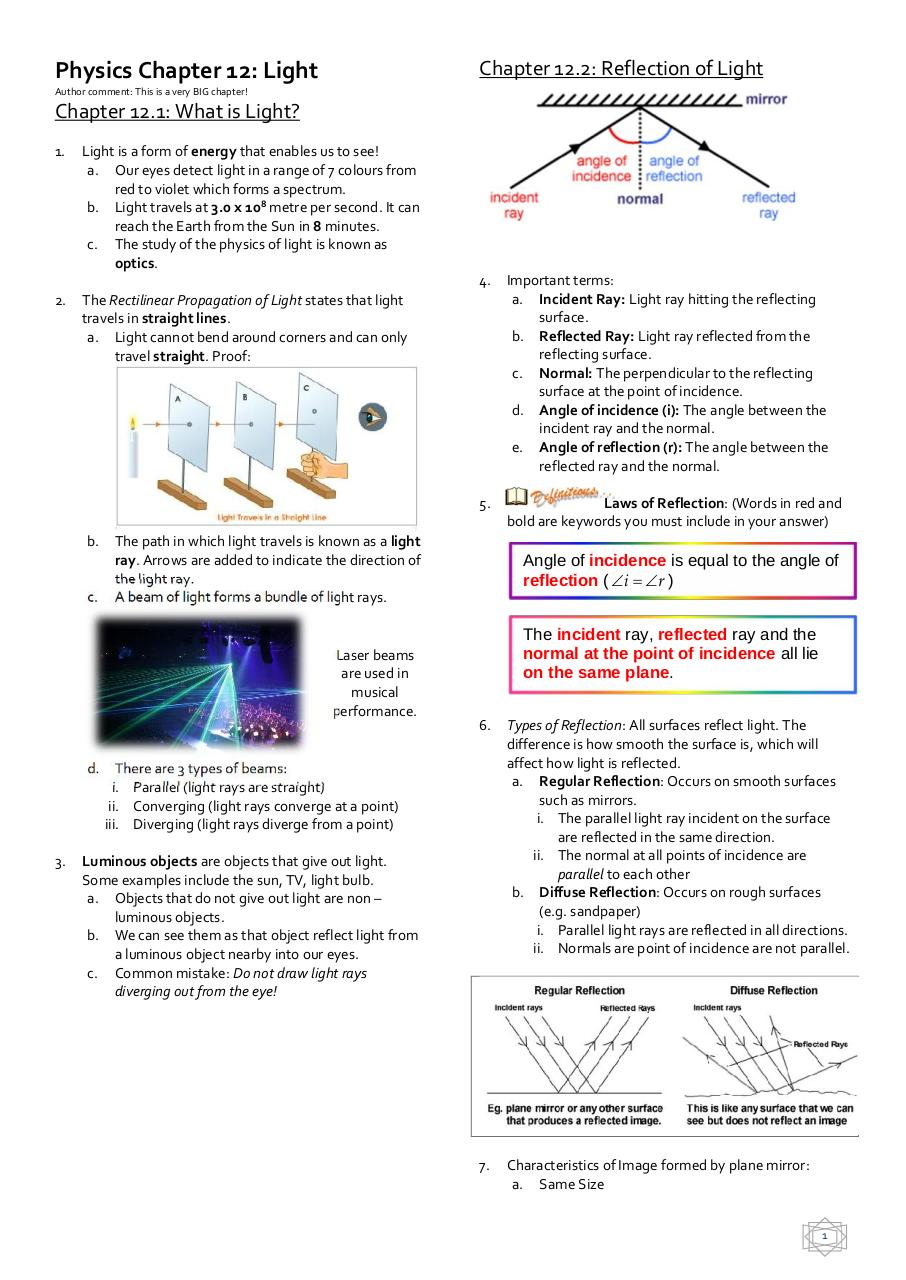# Waves.pdfPage 1 2 3 4 5 6 7

#### Text preview

Physics Chapter 12: Light

Chapter 12.2: Reflection of Light

Author comment: This is a very BIG chapter!

Chapter 12.1: What is Light?
1.

2.

Light is a form of energy that enables us to see!
a. Our eyes detect light in a range of 7 colours from
red to violet which forms a spectrum.
b. Light travels at 3.0 x 108 metre per second. It can
reach the Earth from the Sun in 8 minutes.
c. The study of the physics of light is known as
optics.

Important terms:
a. Incident Ray: Light ray hitting the reflecting
surface.
b. Reflected Ray: Light ray reflected from the
reflecting surface.
c. Normal: The perpendicular to the reflecting
surface at the point of incidence.
d. Angle of incidence (i): The angle between the
incident ray and the normal.
e. Angle of reflection (r): The angle between the
reflected ray and the normal.

5.

Laws of Reflection: (Words in red and

The Rectilinear Propagation of Light states that light
travels in straight lines.
a. Light cannot bend around corners and can only
travel straight. Proof:

b.
c.

The path in which light travels is known as a light
ray. Arrows are added to indicate the direction of
the light ray.
A beam of light forms a bundle of light rays.

Laser beams
are used in
musical
performance.

d.

3.

4.

Angle of incidence is equal to the angle of
reflection ( i  r )
The incident ray, reflected ray and the
normal at the point of incidence all lie
on the same plane.
6.

Types of Reflection: All surfaces reflect light. The
difference is how smooth the surface is, which will
affect how light is reflected.
a. Regular Reflection: Occurs on smooth surfaces
such as mirrors.
i. The parallel light ray incident on the surface
are reflected in the same direction.
ii. The normal at all points of incidence are
parallel to each other
b. Diffuse Reflection: Occurs on rough surfaces
(e.g. sandpaper)
i. Parallel light rays are reflected in all directions.
ii. Normals are point of incidence are not parallel.

7.

Characteristics of Image formed by plane mirror:
a. Same Size

There are 3 types of beams:
i. Parallel (light rays are straight)
ii. Converging (light rays converge at a point)
iii. Diverging (light rays diverge from a point)

Luminous objects are objects that give out light.
Some examples include the sun, TV, light bulb.
a. Objects that do not give out light are non –
luminous objects.
b. We can see them as that object reflect light from
a luminous object nearby into our eyes.
c. Common mistake: Do not draw light rays
diverging out from the eye!

1